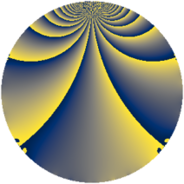# Properties

 Label 3528.1.coLevel $3528$ Weight $1$ Character orbit 3528.co Rep. character $\chi_{3528}(587,\cdot)$ Character field $\Q(\zeta_{6})$ Dimension $16$ Newform subspaces $1$ Sturm bound $672$ Trace bound $0$

# Related objects

## Defining parameters

 Level: $$N$$ $$=$$ $$3528 = 2^{3} \cdot 3^{2} \cdot 7^{2}$$ Weight: $$k$$ $$=$$ $$1$$ Character orbit: $$[\chi]$$ $$=$$ 3528.co (of order $$6$$ and degree $$2$$) Character conductor: $$\operatorname{cond}(\chi)$$ $$=$$ $$504$$ Character field: $$\Q(\zeta_{6})$$ Newform subspaces: $$1$$ Sturm bound: $$672$$ Trace bound: $$0$$

## Dimensions

The following table gives the dimensions of various subspaces of $$M_{1}(3528, [\chi])$$.

Total New Old
Modular forms 48 32 16
Cusp forms 16 16 0
Eisenstein series 32 16 16

The following table gives the dimensions of subspaces with specified projective image type.

$$D_n$$ $$A_4$$ $$S_4$$ $$A_5$$
Dimension 16 0 0 0

## Trace form

 $$16q + 8q^{4} + O(q^{10})$$ $$16q + 8q^{4} - 8q^{16} - 8q^{25} - 16q^{64} + 8q^{99} + O(q^{100})$$

## Decomposition of $$S_{1}^{\mathrm{new}}(3528, [\chi])$$ into newform subspaces

Label Dim. $$A$$ Field Image CM RM Traces $q$-expansion
$$a_2$$ $$a_3$$ $$a_5$$ $$a_7$$
3528.1.co.a $$16$$ $$1.761$$ $$\Q(\zeta_{48})$$ $$D_{24}$$ $$\Q(\sqrt{-2})$$ None $$0$$ $$0$$ $$0$$ $$0$$ $$q-\zeta_{48}^{20}q^{2}+\zeta_{48}^{5}q^{3}-\zeta_{48}^{16}q^{4}+\cdots$$